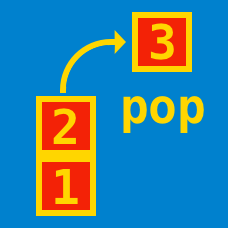Computer Science

# Stacks - Intermediate

Consider the standard algorithm for converting a decimal number (base 10) into a binary number (base 2). This algorithm makes use of a single stack.

How many times will an item be pushed to this stack if the number to be converted is $1048596$?

Given a contiguous histogram each of integer height and unit width, write a program that finds the maximum possible rectangular area.For the histogram above, the maximum area is $6\times4=24$. What is the maximum area for the following data table?Note

There are many ways to approach this question, but an efficient way of solving this is using stacks.

A polish expression or postfix expression is a notational form for logic, arithmetic, and algebra which places operators to the left of their operands. What is the value of the polish expression below when evaluated?

$\large{35\ 17\ 40\ 9\ - * + 7 - }$

×

Problem Loading...

Note Loading...

Set Loading...